Classics in the History of Psychology

An internet resource developed by

Christopher D. Green
York University, Toronto, Ontario

THE INFLUENCE OF IMPROVEMENT IN ONE MENTAL FUNCTION
UPON THE EFFICIENCY OF OTHER FUNCTIONS

II. THE ESTIMATION OF MAGNITUDES

Edward L. Thorndike & R. S. Woodworth (1901)

First published in Psychological Review, 8, 384-395.

In a previous paper we considered in detail a typical experiment on the influence of training in delicate estimation of magnitudes. The present paper will summarize all the experiments of that sort which we have made with individuals.

Before and after training in judging the areas of rectangles 10-100 sq. cm. in size, four subjects were tested as to their ability to judge:

1. Triangles within the same limits of size.
2. Areas between 140-200 sq. cm. of similar shape to those of the training series.
3. Areas between 200-300 sq. cm. of similar shape to those of the training series.
4. Areas between 100-140 sq. cm. of various shapes; circles, trapezoids, etc.
5. Areas of 140-200 sq. cm. of various shapes; circles, trapezoids, etc.
6. Areas between 200-240 sq. cm. of various shapes; circles, trapezoids, etc.
7. Areas of 240 sq. cm. and over of various shapes; circles, trapezoids, etc.

Table IV. represents the results with these subjects. The figures after each name in part A represent the average errors for the kind of area stated at the head of the column, in the before- and after-training tests. In part B are given percent- [p. 385]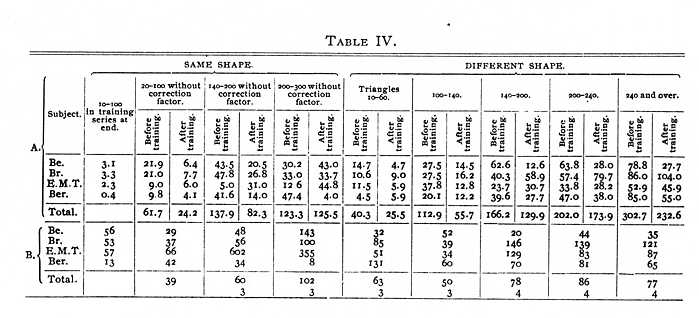[p. 386] ages showing the proportion of the late to the early errors. The percentages after 'total' represent the proportion of the sum of the average errors of all four in the after-training tests to the sum of the average errors in the before-training tests. The figures beneath represent the number who make a smaller proportionate improvement, in the case of each category, than they did in the case of areas exactly similar to those of the training series but estimated without the correction factor, i.e., in just the same way that they estimated the triangles, irregular areas, etc. It has seemed unwise to attempt in detail the calculation of the reliability of each of these and of following results. The labor would be enormous and in many cases the laws of chance not easily applicable. In these preliminary studies we have tried to discover only general tendencies, not their exact amount.

From the figures given for these subjects it seems clear (1) that the improvement in the estimation of rectangles 10 to 100 sq. cm. is not equalled in the other functions; (2) that change in size without change in shape decreases the amount of improvement in proportion, in general, to the amount of the change, and (3) that the same tends to hold true when both size and shape change. The score for areas 240 and over presents an exception to this which cannot, we think, be due to chance. (4) The different influence of the training on the different subjects is apparent from the last column. It teaches, as was pointed out in a previous article, that there is no inner necessity for improvement of one function to improve others closely similar to it, due to a subtle transfer of practice effect. Improvement in them seems due to definite factors, the operation of which the training may or may not secure.

Two subjects took the training in the same manner as did these four but were tested with only parts of the series. Their records were as in Table V.

Experiments similar in the general plan to these were carried on in the case of several other sorts of estimations of magnitude. A detailed account of their administration is out of the question. As has been pointed out, an exact measure of the improvement in the case of the different training series has not [p. 387] been possible. In the following results whenever a measure of such improvement is given, it means the change from the average of the first trial of the whole series to that of the last trial.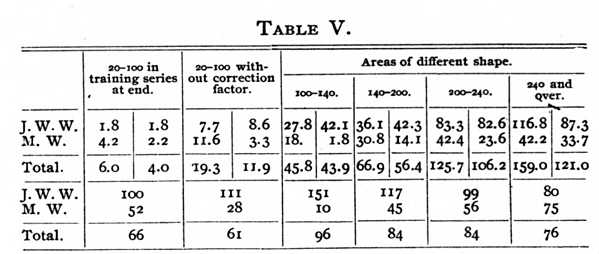The influence of training in estimations of magnitude within certain limits of the ability to estimate similar magnitudes in case of objects qualitatively different.

1. The influence of training in estimating the areas of rectangles from 2 to 12 square inches on the ability to estimate triangles from 1 to 5.5 square inches.

The general method was the same as has been described. The series used for the training was a set of 60 rectangles of various shapes, ranging from 1.5 to 12 sq. inches. It was expected that the number would be so great as to prevent any from being known by their shape, and the records are free from any proof that such was the case. It may have been, however, that the subjects were to some extent unconsciously guided by other factors than the mere magnitudes.

Subject W. in the rectangles series, being allowed to note the real lengths after each judgment, made sum of deviations 30.1 square inches (approx.).

After 20 trials, 5 with about two-thirds and 15 with the whole series, he made 11.5, being 28.3 per cent. of his first trial (average errors approximately .5 and .2). With the tri- [p. 388] angle series W. was tested before and after this training, the results being sums of deviations 2.5 square inches and 5.75 square inches, the latter being 230 per cent. of the former (average errors .11 and .26 square inch). The average error of areas in the training series of corresponding sizes at the end of training was approximately .07 square inch.

Subject T. in a similar way made 30.0 (approximate) at the start and after approximately 20 trials made 39 per cent. of the former (average errors approximately .5 and .2). In tests with the triangle series before and after this training T.'s sums of deviations were 15.0 and 6.5, 43.3 per cent. of the former. Average errors were .68 and .30. The average error for areas in the training series of corresponding size was at the end of training .07 square inch approximately.

2. The influence of training in estimating the areas of rectangles and triangles from 0.5 to 12.0 square inches on the ability to estimate various shapes between the same limits.

The general method was the same that has been described. The series used for the training was the set of rectangles used in 1, plus 42 triangles of different shapes, ranging from 1.5 to 5.5 square inches by .5 square inch steps.

The note on page 387 is equally applicable here. Before and after this training the subjects were tested with 17 areas of various irregular shape running from 3.1 square inches to 11.8, and averaging 6.4.

Subject W., starting from the point of ability given by experiment 2, and being allowed to note the real lengths after each judgment, made in the first trial sum of deviations 21 square inches, at the end of 32 trials 8 square inches, 38 per cent. of the former (the training was at intervals of about a week during over a month, hence the slow progress). The average errors were approximately .21 and .08 square inch. Before and after this training he was tested with the irregular shape series, the results being sums of deviations 17.17 and 16.83 square inches, or 98.0 per cent. of the former. Average errors, [p. 389] 1.01 and .99. The average error for corresponding sizes in training series was at the end of training approximately .2.

Subject T. in a similar manner made in the first trial sum of deviations 26.5 square inches, at the end of 41 trials 9.0 square inches, 34 per cent. of the former (the training was over a similar time to W.'s). The average errors were approximately .26 and .09. Before and after this training his results with the irregular shape series were sums of deviations 34.1 and 11.7, the latter being 31.3 per cent. of the former. Average errors 2 and .69. The average error for corresponding sizes in the training series was at the end approximately .2.

Subject N. was tested with the same series as W. and T., but estimated the areas in square centimeters. She was trained with a series of rectangles of 20 to 60 sq. cm. varying each from the next by one sq. cm., there being two of each size. With the 20-60 sq. cm. series, being told only that the limits were 20 and 60 cm. and that 1 inch equalled 2.54 sq. cm., N. made an average error of 4 sq. cm. Being then allowed to note the real area after each judgment, she made in her first trial with the series an average error of 2.2 sq. cm. At the end of 28 trials her average error was 0.55 sq. cm., 14 per cent. of the first error, 25 per cent. of the second.

Before any knowledge save that 1 inch equalled 2.54 cm., N. made with estimates of ten of the test series an average error of 63.0 sq. cm., the average real size being 122.3. Of these, four were under 60 sq. cm., averaging 38.4. The average error for these four was 22.7. After two minutes' observation of a sq. cm., a 10 sq. cm., a 50 sq. cm. and a 100 sq. cm. area, N. made for these four (when mixed in the total series) an average error of 8.8. For the series of varied shapes (12 being used) she made under similar circumstances average error 12.4, sum of deviations 148.6. After the 28 trials with the training series her average error was 3.6, sum of deviations 44.8, 30 per cent. of the former. For the four areas previously mentioned her average error was 6.0. In brief, her improvement due to [p. 390] the slight chance to acquire a standard was nearly twice that due to the actual training, in so far as the four determinations were a fair test. For areas in the training series of sizes corresponding to the varied shapes of the test series her average error at the end of training was approximately .6.

3. The influence of training in estimating weights of 40 to 120 grams on the ability to estimate the weights of miscellaneous objects of similar weights.

The test weights were eight in number, averaging 95.8 grams. The objects were a cup, umbrella handle, pack of cards, etc.

The training was of the general method described, a series of weights 40, 45, 50, 55, * * * 120 grams, differing no wise save in weight, being used.

Subject W., being allowed from the start to note after each judgment the correct weight, made at his first trial with the series sum of deviations 245, after 50 trials with the series sum of deviations 125. Average errors, 14 and 7.

In tests with the eight weights before and after this training he made sum of deviations 377 and 142, the average errors being 47 and 18. Six judgments improved, 2 were worse. With corresponding weights of the training series the average error at the end of training was 9.

Subject T. in a similar experiment made at his first and last trials (T. took 100 trials) with the 40-120 series sums of deviations 135 and 80, 59 per cent. of the former.

In tests with the eight weights before and after this training T. made sums of deviations 182.5 and 159.5, 87 per cent. of the former, the average errors being 22.6 and 19.9. Three judgments improved, 5 were worse. With corresponding weights of the training series the average error at the end of training was 3.

The influence of training in estimations of magnitude within certain limits on the ability to estimate magnitudes outside those limits.

1. The influence of training in estimating lengths from .5 to 1.5 inches on the ability to estimate lengths of 6.0 to 12.0 inches.

[p. 391] The training was of the general type described on page 250, the series used being a set of cards on each of which was drawn a line. The series contained 5 lines of 1/2 inch and 4 lines of each of the following sizes, 5/8, 3/4, 7/8, etc., up to 1 1/2. The subject was permitted from the start to note after each judgment the real value. In W.'s case the sum of deviations for the first trial was 9 (eighths of an inch). In the last of 40 trials it was 2. The inaccuracy in the last trial was thus 22 per cent. of that in the first.

Before the 1st and after the 40th trial, W. estimated the lengths of 28 lines from 6 to 12 inches long. His sums of deviations before and after the training were 7.5 inches and 11 inches respectively, the number of errors being 13 and 19.

Subject T. in a similar experiment made with the training series in the first trial sum of deviations 8 (the average of the first three trials was 10 1/3). In the last of 24 trials the sum of deviations was 2 (the average of the last three trials being 1 2/3). The inaccuracy of the last trial was thus 25 per cent. of that of the first. With the test series T. made sums of deviations 7.5 and 7.5, the number of errors being 15 and 14.

For four other subjects the records were as follows: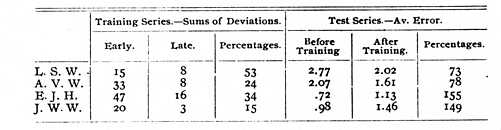2. The influence of training in estimating lengths of 6.0 to 12.0 inches on ability to estimate lengths of 15 to 24 inches.

The method of the experiment was the same as in 1. The series used for the training was 20 lines from 6 to 12 inches long. The series used for the tests was 13 lines from 15 to 24 inches long.

Subject C. in the 6-12 inch series made sum of deviations 40 when estimating the lengths without aid, save the knowledge that they were between 6 and 12. In the next trial, being allowed to note the real length after each judgment, she made [p. 392] sum of deviations 23. After 40 trials with the set (roughly 80 units of trial) her sum of deviations was 5.6, 24.3 per cent of her second trial, 14 per cent. of her first trial. With the 15-24 inch series she was tested before the 1st and after the 40th, the results being sums of deviations 31 (all minus) and 9 (8-, 1+), the latter being 29.0 per cent. of the former.

Subject N., being allowed to note the real lengths after each judgment, made sum of deviations 23. After 32 trials (roughly 64 units of trial) her sum of deviations was 1.0 (approximately), 4.0 per cent. (approximately) of her first trial. With the 15-24 inch series she was tested after the 8th and 32d, the results being sums of deviations 16 and 13, the latter being 81.0 per cent. of the former. During the period from trial 8 to trial 32 her improvement on the 6-12 inch lines was such as to reduce the sum of deviations from approximately 10 to approximately 1, that is, to 10.0 per cent.

The influence of training in estimations of magnitudes within limits on the ability to estimate magnitudes outside those limits, the objects being in addition qualitatively different.

1. The influence of training in estimations of areas of rectangles and triangles of 0.5 to 12.0 square inches on the ability to judge areas from 12 to 65 square inches of different sorts of shapes.

The training series has been described on page 388. The test series contained 10 areas 12 to 18 inches, averaging 14.1, 6 areas 18 to 24 square inches, averaging 20.9, 6 areas 24 to 30 square inches, averaging 28.5, 5 areas 30 to 36 square inches, averaging 34.1, 11 areas 36-65 square inches, averaging 44.5.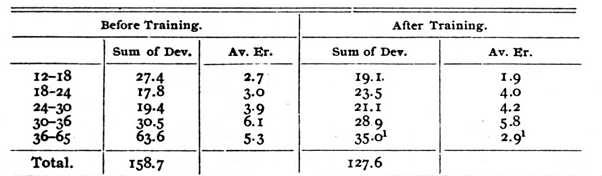[p. 393] Subject W. was tested before and after the training described on page 388. The results were as shown on previous page.

The total error after training was thus 80 per cent. of that before training.

Subject T. was tested before and after the training described. The results were: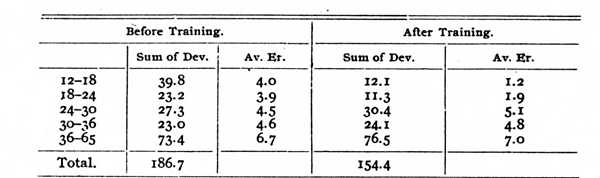The total error after training was thus 83 per cent. of that before training.

Subject N. was tested with the large areas at the same times and in the same manner as described on page 389, before and after the training there described. Her estimates were made in square centimeters. Dividing the areas used in the test into those between 60 and 100, 100 and 140, 140 and 200, 200 and 240, and 240 and over we get the following results: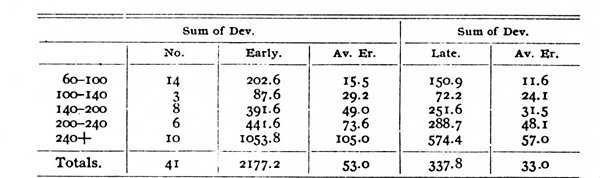The inaccuracy in the late tests was thus 61 per cent. of that in the former.

Before any knowledge save that 1 inch equals 2.54 cm., N. made with 10 of the test series an average error of 63 sq. cm. Of these, 6 were above 60 sq. cm., averaging 178 sq. cm. Her average error for these was 90.1. After two minutes' observations of a sq. cm., a 10 sq. cm., a 50 sq. cm. and a 100 sq. cm. area, N. made for these six (when mixed in the total series) an average error of 55.5, 62 per cent. of the former.

[p. 394] 2. The influence of training in estimating weights of 40 to 120 grams on the ability to estimate the weights of miscellaneous objects of weights outside 40-120 grams.

The test weights were 12 in number, averaging 736 grams. The objects were books, a shoe, a bottle, etc.

The training was that described on page 390.

W. was tested before and after the training with the 40-120 series. The sums of deviations were 1438 and 958, the average errors being 120 and 80. Of the 12, six estimations were improved, two equal, four worse. One case of improvement was from 390 to 90.

T., in a similar experiment with training as described on page 390, made before and after training sums of deviations 1128 and 1142, the average errors being 94 and 95. 6 judgments improved, three were equal and three worse.

3. The influence of training in estimating lengths of lines from 0.5 to 1.5 inches on the ability to estimate the lengths of objects qualitatively different of 2.5 to 8.75 inches.

Subject W. before and after the training described on page 391 was tested with 12 objects, e.g., an envelope, a brush, a wrench, the average length being 5.8 inches. His sums of deviations were 5.0 and 5.0, being the same. The average error was 0.42 -- in both cases.

Subject T. in a similar experiment made with a series of ten such objects of nearly the same average length, sums of deviations 2.75 and 3.25, the average errors being 0.275 and 0.325.

When one undergoes training in estimating certain magnitudes he may improve in estimating others from various causes. Such training as was described in our previous paper gives one more accurate mental standards and more delicacy in judging different magnitudes by them. In the case of estimations of magnitudes in terms of unfamiliar standards such as grams or centimeters, the acquisition of the mere idea of what a gram or centimeter is, makes a tremendous difference in all judgments. This will be seen in the case of N.'s estimation of areas. She was told that an inch was 2.54 centimeters, and with that as practically the sum of her knowledge of the size of a centimeter [p. 395] made judgments of a certain inaccuracy. The mere examination for two minutes of areas 1, 10, 50 and 100 sq. cm. in size reduced this inaccuracy to 38 per cent. of what it had been. The acquisition of definite ideas is thus an important part of the influence of improvement in one function on the efficiency of other functions. Even this, however, may not be operative. With some subjects in some cases the new ideas or the refinements of old ideas produced by the training seem impotent to influence judgments with slightly different data.

It is hard to prove whether or not to what extent the delicacy in judging by means of such ideas in the case of one set of data, is operative with the different data used in the test series. Surely it sometimes is not.

The training might also give ideas of how to most successfully estimate, habits of making the judgments in better ways, of making allowance for constant errors, of avoiding certain prejudices. These habits might often concern features in which the function trained and the functions tested were identical. For instance, the subjects who judged areas of various shapes made their judgments before training by looking at the 10, 25 and 100 sq. cm. areas given them as guides; after training they never looked at these but used the mental standards acquired. This habit is a favorable one, for a person can look at a 25 sq. cm. area in the shape of a square and still think various-shaped areas from 30 to 50 sq. cm. are under 30. The mental standard works better.

The training might give some mysterious discipline to mental powers which we could not analyze but could only speak of vaguely as training of discrimination or attention. If present, such an effect should be widely and rather evenly present, since the training in every case followed the same plan. It was not.

For functions so similar and for cases so favourable for getting better standards and better habits of judging the amount of improvement gotten by training in an allied function is small. Studies of the influence of the training of similar functions in school and in the ordinary course of life, so far as we have made such, show a similar failure to bring large increases of efficiency in allied functions.

Footnotes

 The areas from 8 to 9.5 were added after the 5th trial. The sums of deviations for the first five trials were 16.5, 9.5, 6.5, 8.5, 7. They then rose to 17.5, 25.0, 21, 19.5, etc. By calculating what the sum of deviation would have been had the series been full from the start, we get 30.0 square inches.

 These areas were determined by careful weighing, but their accuracy is conditioned by such slight variations as there were in the thickness of the paper used.

 This series was intended to be made up of areas indistinguishable save by size, but their shapes did perhaps afford some opportunity for unconscious influence on the estimations.

 Save in the first few trials, where 25 per cent. were unduplicated.

 [Classics Ed.: for the two 1s in the table] The notable decrease in error here was due to a few very great improvements. Out of 12 judgments 4 were worse than before training, 1 was the same and 7 were better.